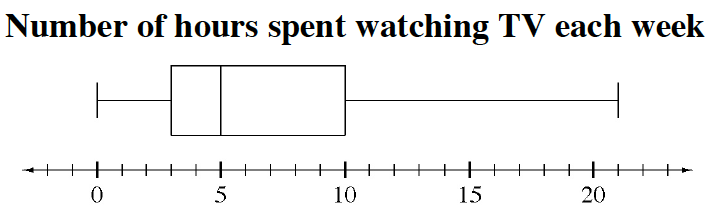### Home > CC3 > Chapter 6 > Lesson 6.2.4 > Problem6-84

6-84.

Kevin found the box plot below in the school newspaper.1. Based on the plot, what percent of students watch more than $10$ hours of television each week?

What is the median?
What are the upper and lower quartiles?

1. Based on the plot, what percent of students watch less than $5$ hours of television each week?

Read the box plot above.

1. Can Kevin use the box plot to find the mean (average) number of hours of television students watch each week? If so, what is it? Explain your reasoning.

Can you find the mean if the individual data points are not given?